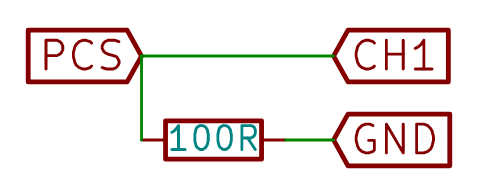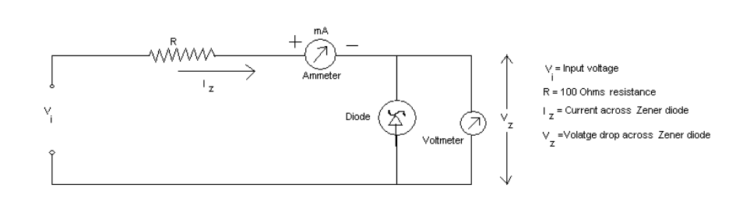## Implementing Experiment Functionality in PSLab Android

Using the PSLab Hardware Device, users can perform experiments in various domains like Electronics, Electrical, Physics, School Level experiments, etc. These experiments can be performed using functionalities exposed by hardware device like Programmable Voltage Sources, Programmable Current Source, etc. In this post we will try implementing the functionality to perform an experiment using the PSLab Hardware Device and the PSLab Android App.

Let us take the Ohm’s law experiment as an example and see how it’s implement using the  PSLab Android App.

Ohm’s law states that the current through a conductor between two points is directly proportional to the voltage across the two points, effectively using a constant of proportionality called Resistance (R) where,

`R = V / I`

#### Schematic#### Layout to perform Ohm’s law experiment

The Ohm’s law experiment requires a variable current, so a seekbar is provided to change the current coming from PCS channel, values of which are continuously reflected in the TextView next to it.#### Implementation

The Read button has a listener attached to it. Once it is clicked, the currentValue is updated with the value parsed from the seekbar progress and the selectedChannel variable is assigned from the spinner. These variables are used by the background thread to change the current supplied by current source (PCS pin) of the device and to read the updated voltage from the selected channel of the device.

```btnReadVoltage.setOnClickListener(new View.OnClickListener() {
@Override
public void onClick(View v) {
selectedChannel = channelSelectSpinner.getSelectedItem().toString();
currentValue = Double.parseDouble(tvCurrentValue.getText().toString());
if (scienceLab.isConnected()) {
CalcDataPoint calcDataPoint = new CalcDataPoint();
calcDataPoint.execute();
} else {
Toast.makeText(getContext(), "Device not connected", Toast.LENGTH_SHORT).show();
}
}
});```

CalcDataPoint is an AsyncTask which does all the underlying work like setting the current at the PCS channel, reading the voltage from the CH1 channel and triggering the update of the data points on the graph.

```private class CalcDataPoint extends AsyncTask<Void, Void, Void> {

@Override
protected Void doInBackground(Void... params) {
scienceLab.setPCS((float) currentValue);
switch (selectedChannel) {
case "CH1":
voltageValue = scienceLab.getVoltage("CH1", 5);
break;
case "CH2":
voltageValue = scienceLab.getVoltage("CH2", 5);
break;
case "CH3":
voltageValue = scienceLab.getVoltage("CH3", 5);
break;
default:
voltageValue = scienceLab.getVoltage("CH1", 5);
}
return null;
}

@Override
protected void onPostExecute(Void aVoid) {
super.onPostExecute(aVoid);
updateGraph();
}
}```

updateGraph() method is used to update the graph on UI thread. It creates a new dataset from the points which were added by the background thread and refreshes the graph with it using the invalidate() method.

```private void updateGraph() {
tvVoltageValue.setText(df.format(voltageValue));
List<ILineDataSet> dataSets = new ArrayList<>();
List<Entry> temp = new ArrayList<>();
for (int i = 0; i < x.size(); i++) {
}
LineDataSet dataSet = new LineDataSet(temp, "I-V Characteristic");
dataSet.setColor(Color.RED);
dataSet.setDrawValues(false);
outputChart.setData(new LineData(dataSets));
outputChart.invalidate();
}```

We are planning to add an option to support multiple trials of the same experiment and save each trails for further reference. App flow to perform experiment is based on Science Journal app by Google.

#### Resources

• Article on Ohm’s law and Power on electronics-tutorial
• To know more about Voltage, Current, Resistance and Ohm’s law, head on to detailed tutorial on sparkfun
• Implementation of perform experiment functionality in PSLab Desktop App
Continue Reading Implementing Experiment Functionality in PSLab Android

## Performing the Experiments Using the PSLab Android App

General laboratory experiments can be performed using core functionalities offered by the PSLab hardware device like Programmable Voltage Sources, Programmable Current Source, Analog to Digital Converter, Frequency Counter, Function Generators, etc. In this post we will  have a brief look on a general laboratory experiment and how we can perform it using the  PSLab Android App and the PSLab hardware device.

We are going to take Zener I-V Characteristics Curve experiment as an example to understand how we can perform a general experiment using the PSLab device. First, we will  look at the general laboratory experiment and it’s format. Then we will see how that experiment can be performed using the PSLab Android App and the PSLab Hardware Device.

#### Experiment Format of General Experiment in Laboratory

AIM: In this experiment, our aim is to observe the relation between the voltage and the corresponding current that was generated. We will then plot it to get the dependence.

Apparatus:

• A Zener Diode
• A DC Voltage Supplier
• 100 ohm resistor
• 2 multimeter for measuring current and voltages
• Connecting wires

Theory: A Zener Diode is constructed for operation in the reverse breakdown region.The relation between I-V is almost linear in this case, Vz = Vz0 + Iz * Rz , where Rz is the dynamic resistance of the zener at the operating point and Vz0 is the voltage at which the straight-line approximation of the I-V characteristic intersects the horizontal axis. After reaching a certain voltage, called the breakdown voltage, the current increases drastically even for a small change in voltage. However, there is no appreciable change in voltage accompanying this current change. So, when we plot the graph, we get a curve which is very near to the x-axis and nearly parallel to it until a particular potential value, called the Zener potential, is reached. After the Zener potential Vz value, there will be a sudden change and the graph becomes exponential.

Procedure: Construct the circuit as shown in figure belowNow, start increasing the voltage until a reading in the multimeter for current can be obtained. Note that reading. Now, start increasing the input voltage and take the corresponding current readings. Using the set of readings observed,  construct a V vs I graph. This graph gives us the I-V characteristics. The slope of the curve at any point gives the dynamic resistance at that voltage.

Result: The Characteristic curve has been verified after plotting V-I data points on the graph.

#### Experiment format in PSLab Android App

We have a ViewPager that renders two fragments:

1. Experiment Doc– It consists of information like the Aim of experiment, Schematic, Output screenshot that we will get after the experiment has been performed.
2. Experiment Setup– It consists of the setup to configure the PSLab device. This fragment is analogous to the experiment apparatus of the laboratory.

Below is a gif showing the experiment doc of the Zener I-V experiment which is to be performed using the PSLab device. It consists of a schematic and a screenshot of the output that we get after performing the experiment.

Make the circuit connections on a breadboard as shown in the schematic. After the circuit is complete we need to configure experiment.

To configure the experiment, we give the initial voltage, the final voltage and the step size. After clicking on START EXPERIMENT, the voltage is varied on the PV1 channel from the initial voltage to final voltage by increasing the voltage in step size. At each variation of voltage, the current is calculated by dividing the voltage difference between resistor by its resistance value i.e

`I = ( VPV1 - VCH1 ) / R`

As soon as the initial voltage reaches the final voltage, the experiment stops and data points are plotted on the graph. From the graph we can see the change in the current through a zener diode when the voltage varies across it’s terminals.

The output that was obtained after the experiment is I-V characteristic curve for Zener Diode as shown in the image below.It can be clearly seen that after the breakdown voltage (~0.7V) the  current increases drastically with respect to the  increase in the voltage. After this point, the voltage can be considered  nearly constant unlike the current which varies exponentially.

In the PSLab Android App, there are read-back errors while reading bytes serially from the PSLab Hardware Device. As a result, the data points are not read accurately and an inaccurate plot is generated on the graph as shown in the image below.